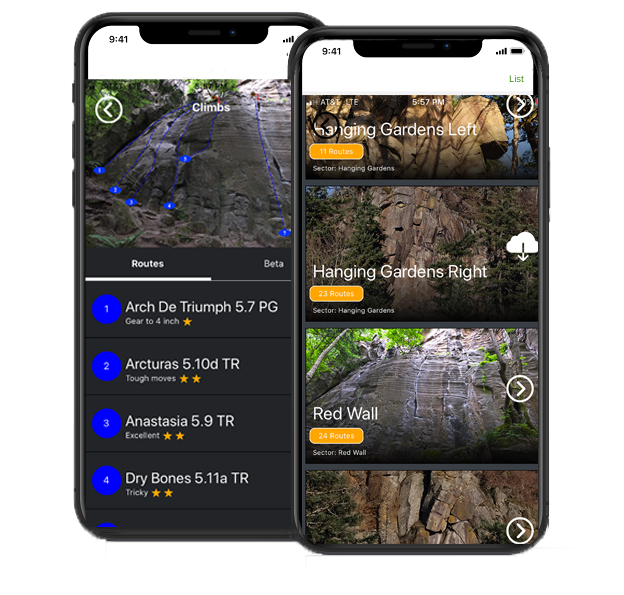Select Page

# Best Rock Climbs

## Best Problems

#### Must-do Classics

This is a test page to add some beta about the boulder problems at Leavenworth.  Below are some boulder problems worth doing and are amongst the most classic and highly traveled problems at Icicle Canyon.  This is a test page to add some beta about the boulder problems at Leavenworth.  Below are some boulder problems worth doing and are amongst the most classic and highly traveled problems at Icicle Canyon. This is a test page to add some beta about the boulder problems at Leavenworth.  Below are some boulder problems worth doing and are amongst the most classic and highly traveled problems at Icicle Canyon.  This is a test page to add some beta about the boulder problems at Leavenworth.  Below are some boulder problems worth doing and are amongst the most classic and highly traveled problems at Icicle Canyon.

## 1. The Dominator V7

This is a test page to add some beta about the boulder problems at Leavenworth.  Below are some boulder problems worth doing and are amongst the most classic and highly traveled problems at Icicle Canyon.

## 2. The Dominator V7

This is a test page to add some beta about the boulder problems at Leavenworth.  Below are some boulder problems worth doing and are amongst the most classic and highly traveled problems at Icicle Canyon.

## 3. The Dominator V7

This is a test page to add some beta about the boulder problems at Leavenworth.  Below are some boulder problems worth doing and are amongst the most classic and highly traveled problems at Icicle Canyon.

## 4. The Dominator V7

This is a test page to add some beta about the boulder problems at Leavenworth.  Below are some boulder problems worth doing and are amongst the most classic and highly traveled problems at Icicle Canyon.

## 5. The Dominator V7

This is a test page to add some beta about the boulder problems at Leavenworth.  Below are some boulder problems worth doing and are amongst the most classic and highly traveled problems at Icicle Canyon.

## 6. The Dominator V7

This is a test page to add some beta about the boulder problems at Leavenworth.  Below are some boulder problems worth doing and are amongst the most classic and highly traveled problems at Icicle Canyon.

Visit the Leavenworth Group## Access Free Guidebook

Join as a basic member to access one free guidebook.